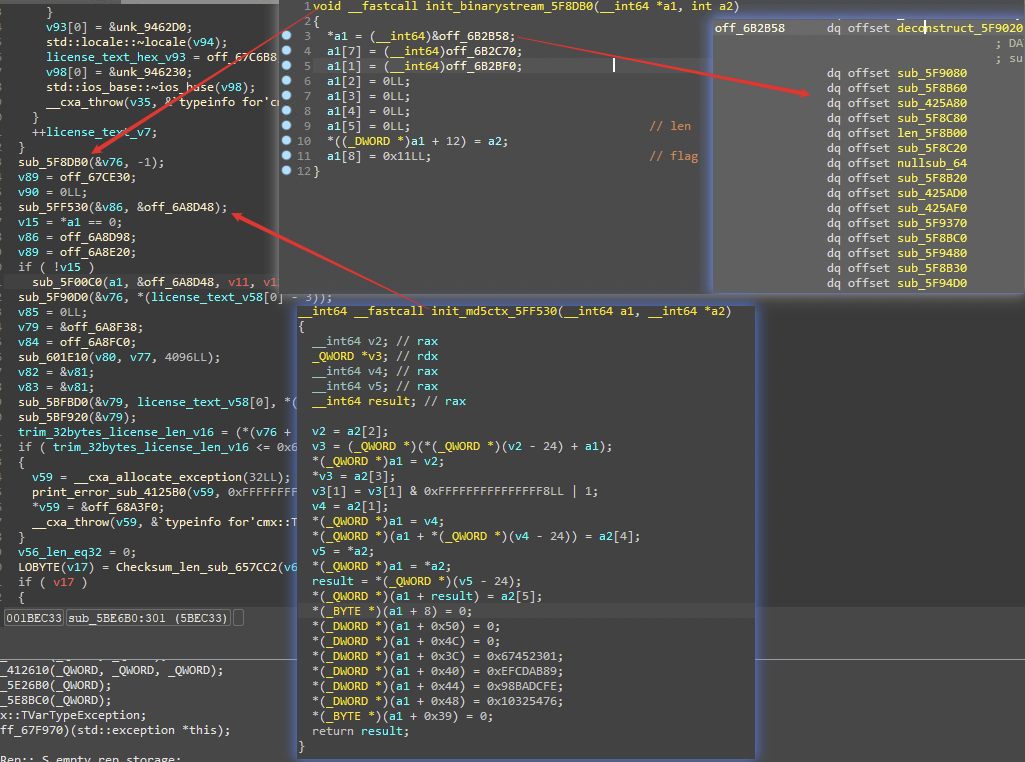# 某CoreMail授权破解分析

Published: 2021年09月21日

In Reverse.

⚠️：这是我第一次逆向无符号C++程序的笔记，但可能并不是一个好的讲怎么逆向C++的例子，以后有时间我会再补上更合适的，现在这个有兴趣就看看吧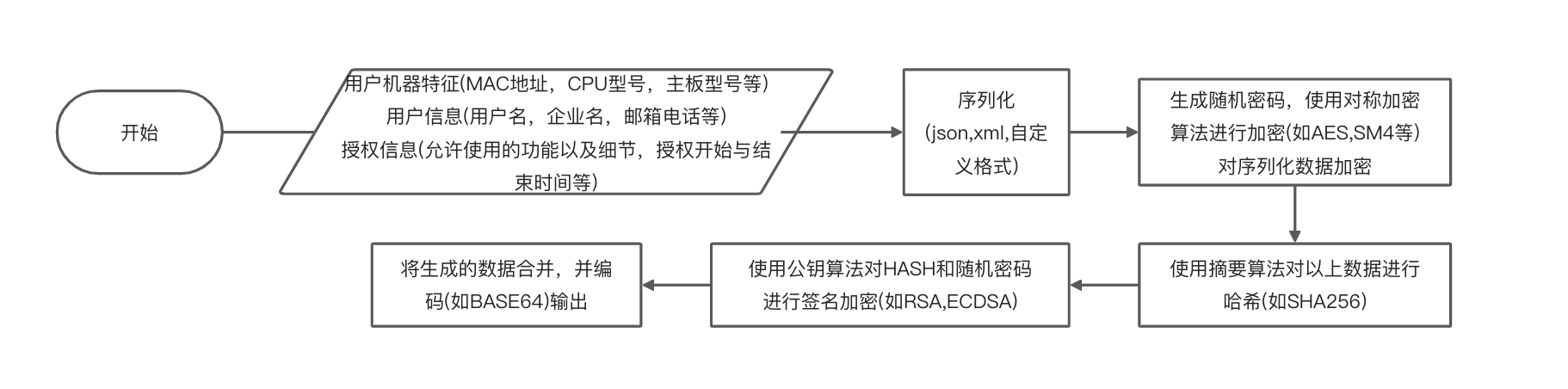1. 输入的证书怎样的格式，它被哪些算法处理过，如签名加密等。
2. 解析后的证书里需要包含哪些内容，如有哪些授权信息，格式是怎样的。

1. 编解码：编解码插件的就那么几种，特征特别明显，如Base64解码存在4变3，这些可参考CTF的杂项题。
2. 摘要：摘要是把任意长度转换为固定长度，如128位，256位，它们一般会有一张常数表。
3. 非对称密码：非对称密码都是用的数学的难题，如大整数分解，椭圆曲线离散对数，特征就是各种大数运算。
4. 对称密码：这里的对称密码也包含序列密码，它们为了雪崩要扩散和混淆，因此会有很多循环，异或的特征。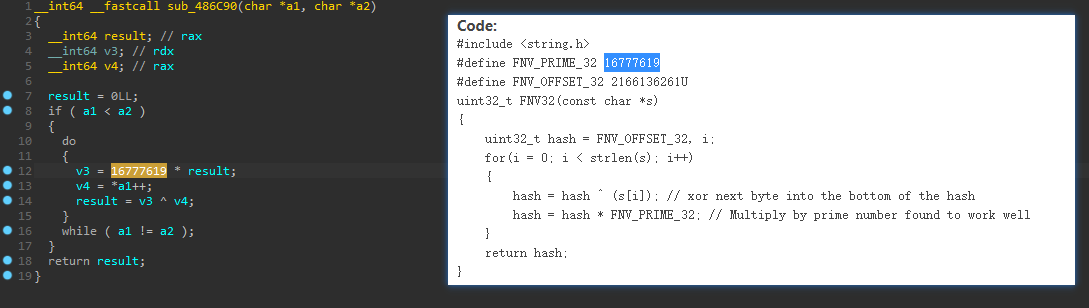1.通过调试，发现上传的证书字符串在`sub_5BE6B0`内被解析，任意输入的数据将会报证书相关异常，分析该函数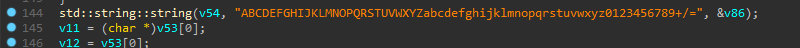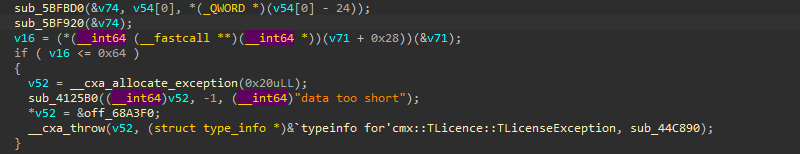2.若满足条件会讲解码后的数据传入如下函数，它的参数4为常数，参数5为一个数组首地址：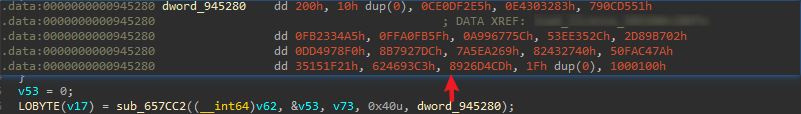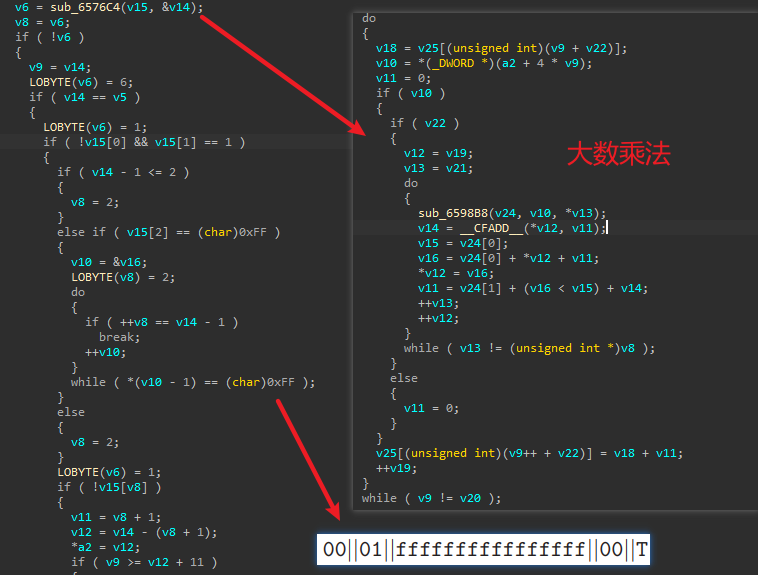``````import gmpy2

RSA_SK = '''-----BEGIN RSA PRIVATE KEY-----
MIIBOgIBAAJBAPEKGpuWnpXu+bfWMM2LpXpZR/R5DhXbCSJBWNLgOlOqCzNws/lj
VE/oOdtsULWuQSmhn4tzXsLZETsRoH73HUMCAwEAAQJAQmiOPB+bQaO9mTCh8X9v
7+15LZnMj6jxM0bdufudFj9SOlO/XRzdgx4Z/uK5D1JrtlSGcgs5OmgrGF+SFtAq
Kpydf/y5KNPVNQkqhVmSpeeFP53jQqECIBVv1ZYYVHCNVfPQzYzumSQYQ8d1NoSX
hKuzeGJKwz9zAiBmXqV2iIJrE4RIVJw1rues/hnGaVCjveHE6COqaJra4QIhALbC
Mwv9nvECYbAM1Gm05eC1p7pARhcfUiK5vWQ9fqv8
-----END RSA PRIVATE KEY-----'''
# openssl genrsa 512
PKCS_MAGIC = bytes.fromhex('0001FFFF FFFFFFFF FFFFFFFF FFFFFFFF FFFFFFFF FFFFFFFF FFFFFFFF FFFFFF00')

def bignum_to_bytes(num: int, pad: int = 0) -> bytes:
"""将大数按大端序转换为字节序列"""
hexstr = hex(num)[2:]
hexstr = '0' + hexstr if len(hexstr) % 2 else hexstr
hex_arr = [int(hexstr[i: i + 2], 16) for i in range(0, len(hexstr), 2)]
hex_arr.reverse()
hex_arr.reverse()
return bytes(hex_arr)

def bytes_to_bignum(data: bytes) -> int:
"""解析大端序存储的大数"""
data_arr = list(map(lambda b: f"{b:02X}", data))
return int(''.join(data_arr), 16)

def rsa512_pkcs_v1_5_encrypt(d: int, N: int, data: bytes) -> int:
"""精简版的加密"""
assert len(data) == 32
M = PKCS_MAGIC + data
M = bytes_to_bignum(M)
return int(gmpy2.powmod(M, d, N))

def rsa512_pkcs_v1_5_decrypt(e: int, N: int, data: bytes) -> bytes:
assert len(data) == 64
C = bytes_to_bignum(data)
M = gmpy2.powmod(C, e, N)
M = bignum_to_bytes(M, 64)
assert M[0:32] == PKCS_MAGIC and len(M) == 64
M = M[32:]
print(bytelist_to_hexstr(M))
return M
``````

3.继续分析`sub_600110`的内容发现为一个初始化函数，根据常数猜测为md5初始化，那么此处为哈希校验：4.继续分析发现做的运算有很多位运算，以及一些轮循环，可以说很像对称加密了（虽然没有找到它的加密盒），而且这里面还有个可疑常数65537，另外之前遇到rsa512这种弱加密了，因此可以怀疑这里使用了一种比较旧的对称加密，搜索发现idea算法完美符合这些特征：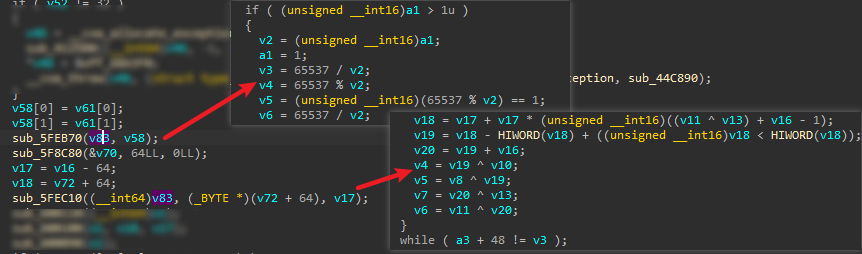``````import ideacipher

BLOCK_SIZE = 8
def idea_decrypt(key: bytes, ciphertext: bytes) -> bytes:
plaintext = b''
for i in range(0, len(ciphertext), BLOCK_SIZE):
block = ciphertext[i:i + BLOCK_SIZE]
if len(block) == BLOCK_SIZE:
plaintext += bytes(ideacipher.decrypt(block, key))
else:
for b in block:
plaintext += struct.pack('<B', (b ^ 0xc5) & 0xff)
return plaintext

def idea_encrypt(key: bytes, plaintext: bytes) -> bytes:
cipher = b''
for i in range(0, len(plaintext), BLOCK_SIZE):
block = plaintext[i:i + BLOCK_SIZE]
if len(block) == BLOCK_SIZE:
cipher += bytes(ideacipher.encrypt(block, key))
else:
for b in block:
cipher += struct.pack('<B', (b ^ 0xc5) & 0xff)
return cipher
``````

5.之后对解密后的数据进行了解析，通过分析得出它将一种类似json的格式解析为c++里的map，因此需要先分析它的自定义格式，如下为处理过的伪代码，在进行一些头部校验后，它会按字节读取，根据类型进入不同的分支进行处理，反序列化为相应的对象：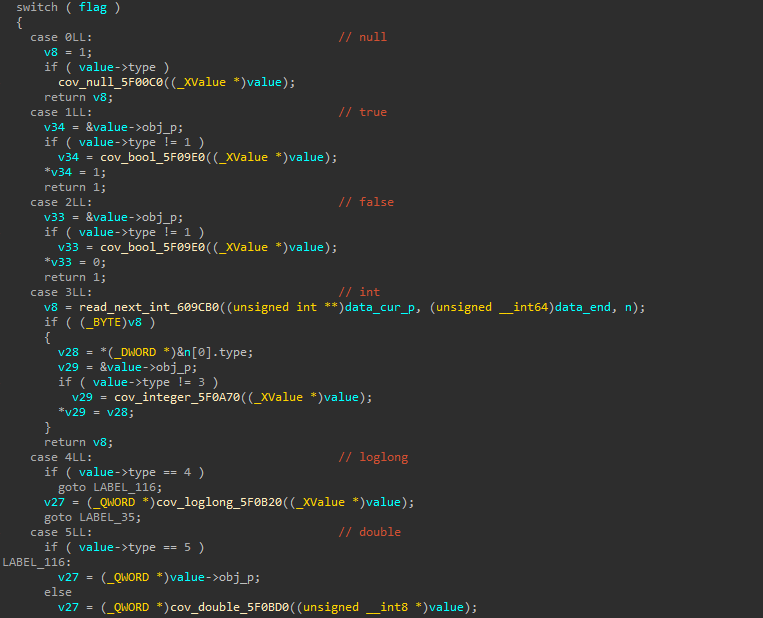``````def pack_str(s: str) -> bytes:
"""将字符串打包"""
num = len(s)
assert num < 0xffffffff
if num < 0xff:
return struct.pack('<B', num) + s.encode()
else:
return b'\xff' + struct.pack('>I', num) + s.encode()

def seralize_node(node) -> bytes:
out = b''
if isinstance(node, dict):
out += b'\x0c'
out += struct.pack('<B', len(node))
for k, v in node.items():
out += pack_str(k)
out += seralize_node(v)
elif isinstance(node, (list, tuple)):
for v in node:
out += seralize_node(v)
elif isinstance(node, str):
out += b'\x06'
out += pack_str(node)
elif isinstance(node, int):
out += b'\x03'
out += struct.pack('>I', node)
elif isinstance(node, DateTime):
out += b'\x0a'
out += node.seralize()
elif isinstance(node, bool):
if node:
out += b'\x01'
else:
out += b'\x02'
else:
raise NotImplemented('S')
return out

def seralize2(json_data):
data = struct.pack('>IHH', 0xffffffff, 0x01, 0x02) + seralize_node(json_data)  # flag + type + version
return data
``````

6.最后，需要分析它要返回的字典应该包含哪些内容，这就要反过来追踪哪些函数会使用此处返回的数据了，最终可得到如下格式，分析完成，可以写注册机了，首先生成合法的RSA参数，生成公钥并替换原始文件：

``````sed -bi 's/...(原始公钥).../\xF1\x0A\x1A...'
``````

``````def gen_lic(json_data):
# 序列化数据
data = struct.pack('>I', 0xffffffff) + seralize2(json_data)
print('证书内容：', data)
# 计算MD5
checksum = md5()
checksum.update(data)
checksum = checksum.hexdigest()
print('校验和：', checksum)
checksum = hexstr_to_bytelist(checksum)
# 对称加密
idea_key = '000100020c024544000100020c024544'
idea_key = hexstr_to_bytelist(idea_key)
data = idea_encrypt(idea_key, data)
print('密文：', bytelist_to_hexstr(data).replace(' ', ''))
# 非对称加密
rsa_plaintext = idea_key + checksum
RSA_KEYPAIR = RSA.importKey(RSA_SK)
N = RSA_KEYPAIR.n
e = RSA_KEYPAIR.e
d = RSA_KEYPAIR.d
rsa_cipher = rsa512_pkcs_v1_5_encrypt(d, N, rsa_plaintext)
print('RSA N: ', bytelist_to_hexstr(bignum_to_bytes(N, 64)))
print('RSA e: ', bytelist_to_hexstr(bignum_to_bytes(e, 32)))
print('RSA d: ', bytelist_to_hexstr(bignum_to_bytes(d, 32)))
data = bignum_to_bytes(rsa_cipher, 64) + data
# base64编码
data = base64.b64encode(data)
print('证书: ', data.decode())
print('编码后证书: ', quote_from_bytes(data, safe=''))
``````